NCERT Solutions for Class 7 Maths Chapter 4 Simple Equations Ex 4.3 are part of NCERT Solutions for Class 7 Maths. Here we have given NCERT Solutions for Class 7 Maths Chapter 4 Simple Equations Ex 4.3.

 Board CBSE Textbook NCERT Class Class 7 Subject Maths Chapter Chapter 4 Chapter Name Simple Equations Exercise Ex 4.3 Number of Questions Solved 4 Category NCERT Solutions

## NCERT Solutions for Class 7 Maths Chapter 4 Simple Equations Ex 4.3

Question 1.
Solve the following equations:Solution: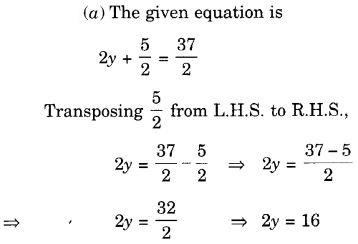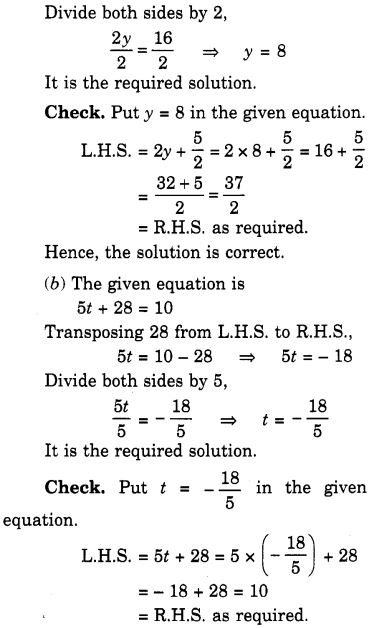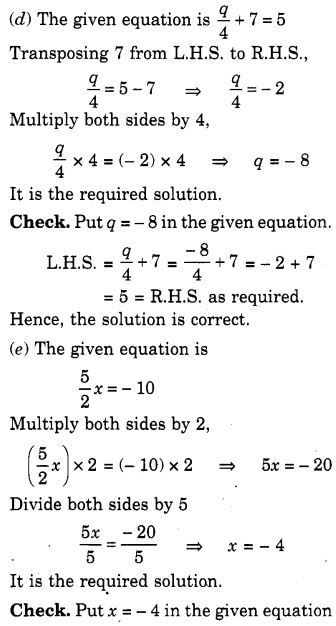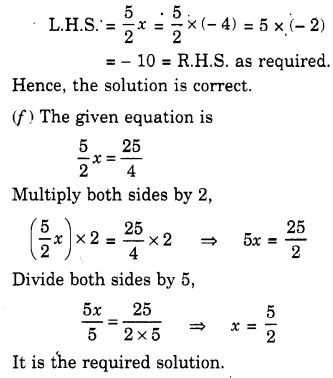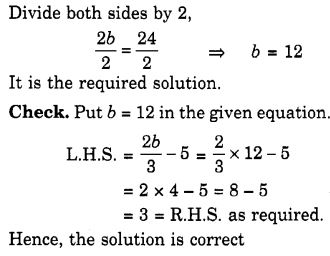Question 2.
Solve the following equations:

(a) 2 (x + 4) = 12
(6) 3 (n – 5) = 21
(c) 3 (n – 5) = -21
(d) -4 (2 + x) = 8
(e) 4 (2 – x) = 8

Solution: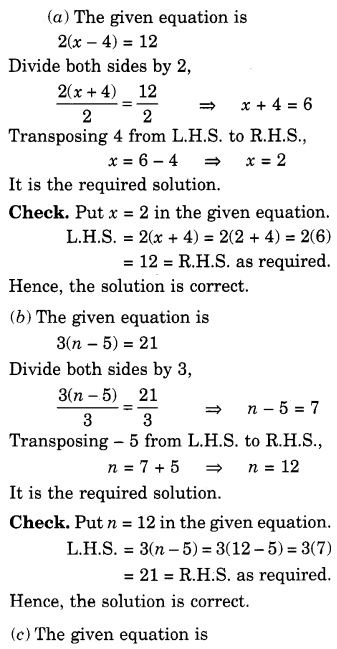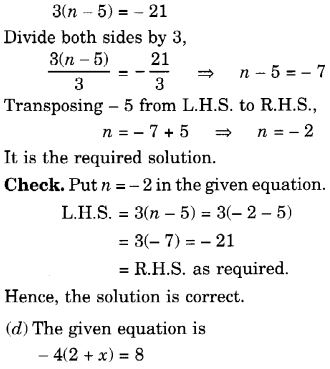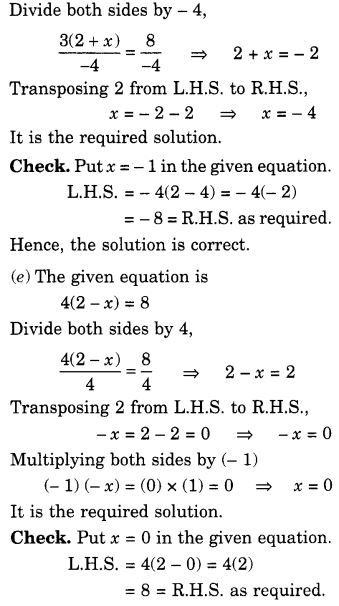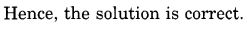Question 3.
Solve the /bilowing equations:

(a) 4 = 5 (p – 2)
(b) -4 = 5 (p – 2)
(c) 16 = 4 + 3 (t + 2)
(d) 4 + 5 (p – 1) = 34
(e) 0 = 16 + 4 (m – 6)

Solution: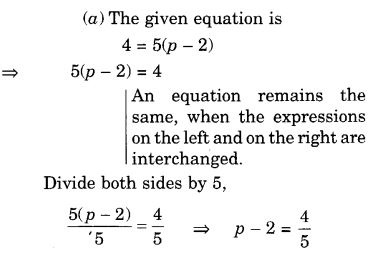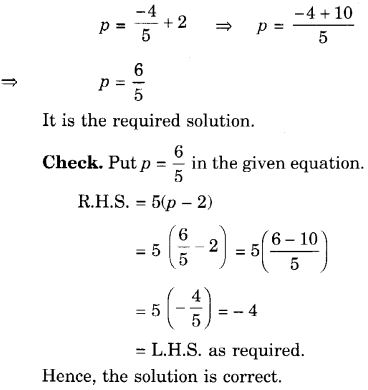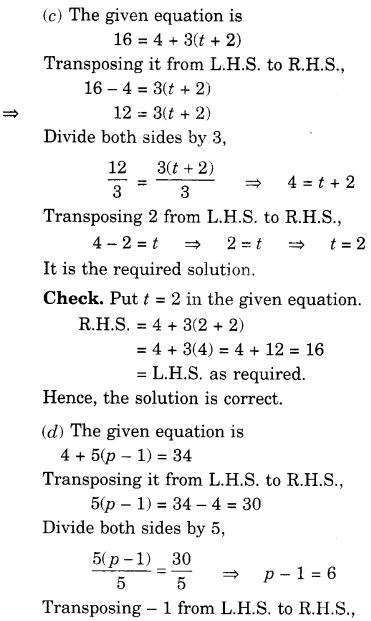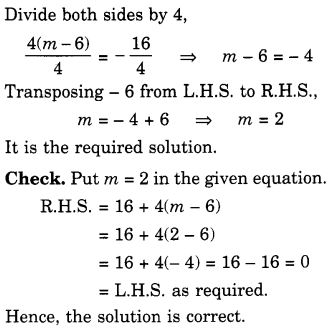Question 4.
(a) Construct 3 equations starting with x = 2
(b) Construct 3 equations starting with x = – 2.
Solution:
Multiply both sides by 3, 3x = 6
Subtract 2 from both sides, 3x – 2 = 4 …(1)

Multiply both sides by 4, 4x = 8
Add 5 to both sides, 4x + 5 = 13 …(2)

3. Start with x = 2 Multiply both sides by 5 5x = 10
Subtract 1 from both sides, 5x – 1 = 9 …(3)

(b) First equation:
Multiply both sides by 2, 2x = -4
Subtract 3 from both sides, 2x – 3 = -7

Second equation:
Divide both sides by 2, $$\frac { x }{ 2 }$$ = -1
Add 3 to both sides, $$\frac { x }{ 2 }$$ + 3 = 2